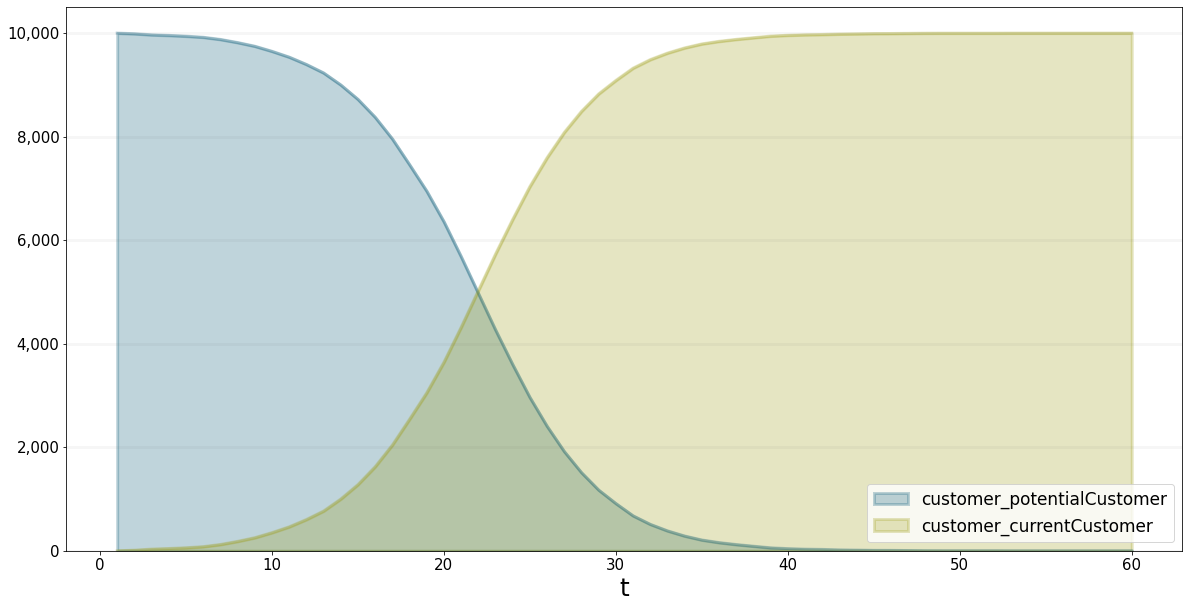Agent-based model of the Bass Diffusion Model

# Bass Diffusion Model

An Agent-based Implementation

This is an implementation of the Bass Diffusion model as an agent-based model using the BPTK_PY framwork. It takes some time to run, hence we use a progress bar.

``````## BPTK Package
from BPTK_Py.bptk import bptk

bptk = bptk()``````
``````bptk.plot_scenarios(
scenario_managers=["ABMsmBass"],
kind="area",
scenarios=["scenarioBassBase"],
agents=["customer"],
agent_states=["potentialCustomer","currentCustomer"],
progress_bar=True
)``````